Popular tags: Support, Resistance, Trend, Range, Fibonacci, Wave, Divergence, Multi time frames(MTF), Swing, Volume, Overbought, Oversold

Featured

# Probability Cone backtest indicator for Thinkorswim

Availability: In Stock
Price: \$150.00USD \$120.00USD
Qty:     - OR -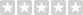(0/5)  0 reviews  |  Write a review
VIP price: \$100

The Probability Cone indicator uses statistical data to predict future price range with specified probability and implied volatility.
It plots a standard deviation curve designating ranges within which prices are predicted.

Thinkorswim has a Probability Analysis tool which is very popular for option trading.

It also has a chart study (ProbabilityOfExpiringCone) that can plot Probability Cone on the most recent bar.

The limitation of the Probability Cone provided by Thinkorswim is that we can only view the cone on the last bar.
It is impossible to know the relative position of current price and previous Probability Cones.

The purpose of this indicator is to give us the ability to back test the Probability Cone.

Features and inputs.

1. Probability: The probability with which price will stay within the calculated range.
2. Bar: the number of bars used to shift backward.
3. VOLATILITY_TYPE: there are 3 options, ImplVol, IMP_VOLATILITY and HistoricalVolatility.
1. ImplVol, this will be the manually input value.
2. IMP_VOLATILITY, this will use the value from IMP_VOLATILITY function.
3. HistoricalVolatility, this will use the value from HistoricalVolatility function.
4. ImplVol: the manually input value of implied volatility to calculate the cone. this input only works if you chose ImplVol as the VOLATILITY_TYPE.
5. basis, this input only works if you chose HistoricalVolatility as the VOLATILITY_TYPE.
6. It only works on Daily, Weekly and Monthly timeframes.
7. It works for all kind of securities, not only Stock, but also Forex and Futures.

If you chose IMP_VOLATILITY or HistoricalVolatility as the VOLATILITY_TYPE, the indicator will use the corresponding value automatically.
As you may already knew that the ImpVolatility on TOS doesn't work for FX, so you can either use the
HistoricalVolatility which will make the indicator to plot based on the corresponding value automatically, or you can
manually input value of implied volatility to calculate the cone.

How it works.

For example, if we have Probability=68.3, IV=0.11 and Bar =20.

The Probability Cone will be calculated and plotted from 20 bars back of the last bar on chart.

Normally, the Probability Cone is used for stock options analysis, but we can also use it in Forex.

The chart shown in below is a perfect example of applying it on FX.

When I test this indicator, I noticed that sometimes we can get better results if we plot the cone from previous swing high and low.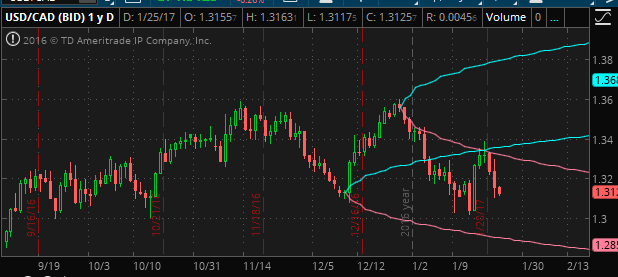The extension lines of two Cones may form a support and resistance zone.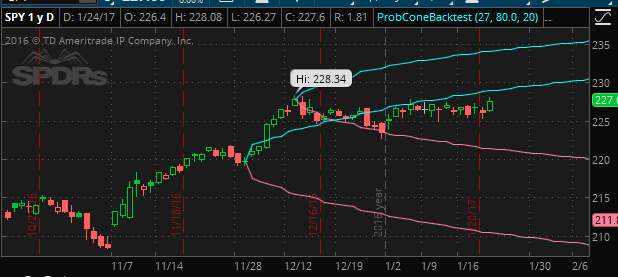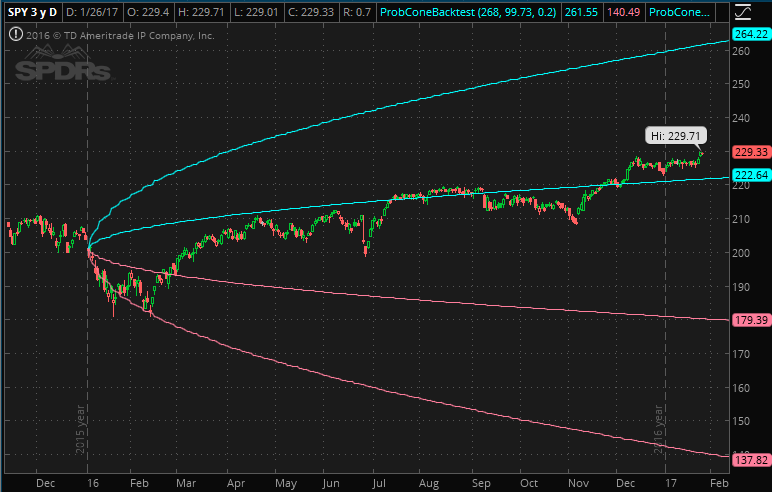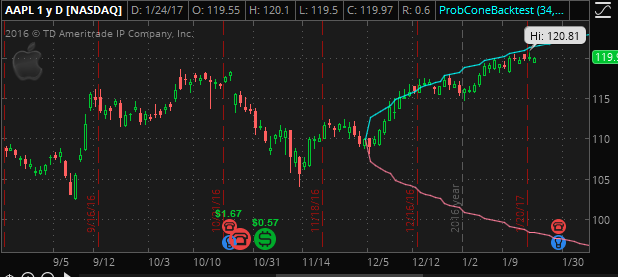## Write a review

Your Review: Note: HTML is not translated!

Enter the code in the box below:Latest
Welcome to Patternsmart
Converting your indicator from one platform to another.